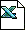## Software Tools and Templates

Robust Systems and Strategy provides here software calculators, templates and tools for free distribution.  No correctness or fitness for use guarentees are provided or implied.

 Sigma capability, Cp, and DPMO Capability Calculator: determine your defect rate, Cpk, or Sigma Capability.Anderson-Darling Normality Test Calculator: check whether your sample data comes from a normally distributed population.Mean (T-test) Sample Size Calculator: determine sample size for ensuring a population average or the difference between two populationsVariance Sample Size Calculator: determine sample size for ensuring a population standard deviation - one or two populationsDefective Proportion Sample Size Calculator - one, two or n populations.  How many samples to assure a maximum defective proportion.Defect Rate per Interval Sample Size Calculator - one or two populations.  How many samples to assure a maximum defect rate.T-Test Sample Size Trade-Off Calculator: a more advanced version of the t-test sample size calculator with ability to trade-off power and confidencet-distribution: how to use Excel's TDIST and TINV for t-distribution calculations.Tolerance Interval Calculator: calculate the required tolerance interval to ensure a quality level based upon a given sample of dataReal Win Worth TemplateCustomer Selection Matrix TemplateCustomer Interview TemplateCustomer Needs List TemplatePugh Concept Evaluation TemplateWeighted Sum Selection Calculator, complete with Error AnalysisQFD TemplateFMEA TemplateKepner-Tregoe PPA TemplateXPI Design-for-Assembly Analysis TemplateStanford Design-for-Assembly Template (from Stanford)Critial Parameter Management Worksheet TemplateDMAIC Six Sigma: Cause and Effect Matrix TemplateDMAIC Six Sigma: Control Plan TemplateDMAIC Six Sigma: KPOV Summary Sheet Template